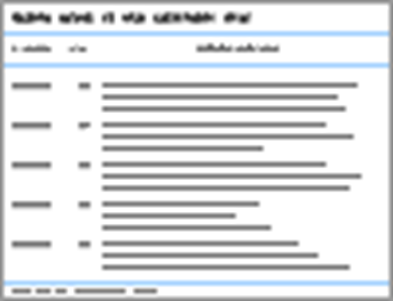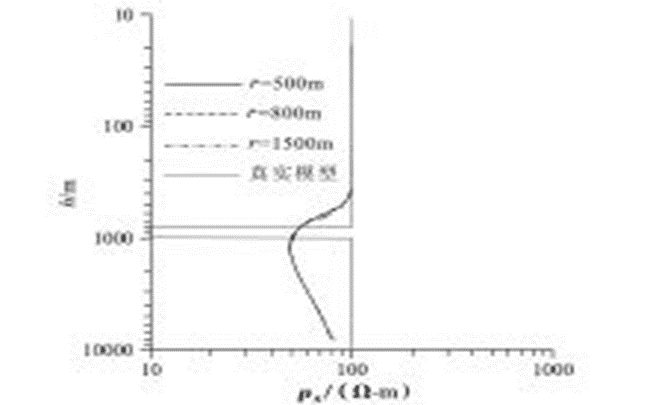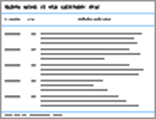Ground Water Source Depth analysis and application of electrical source short offset transient electromagnetic detection

# Ground Water Source Depth analysis and application of electrical source short offset transient electromagnetic detection.## Abstract

There are several concepts of depth in transient electromagnetic method, including diffusion depth, limit detection depth, effective detection depth and apparent detection depth. In this paper, we conducted the calculation, analysis and application to these different depths based on the SME The diffusion depth represent the diffusion and propagation of the underground transient EM field, the limit detection depth reveals the detectability of SOTEM and the results indicate that the greatest depth will be obtained when the offset approximately equal to the 0.7~1 times of buried depth of targets, and the apparent detection depth can give an estimation of the actual detection depth for a given recording time which have a significant meaning to the field work designing. At last,We proposed a synthetic model and a filed example to verify the feasibility and accuracy of using apparent detection depth to estimate the actual detection depth of SOTEM.

Keyword : electrical source ; short-offset ; transient electromagnetic method ; detection depth

## 0 Preface

Apparent resistivity and detection depth are the two most important parameters in electrical prospecting, and detection depth is one of the main criteria to measure the detection ability of a method. Different types of electrical devices have different depth concepts and estimation methods. The direct current method is mainly determined according to the geometric arrangement of its receiving and transmitting devices 1 ] ; in the frequency domain electromagnetic method, the skin depth formula is often used to estimate the detection depth of different frequencies 2 ] ; the time domain electromagnetic method (transient electromagnetic method) involves There are many concepts of depth, such as diffusion depth, limit detection depth, effective detection depth and apparent detection depth

. Of course, in addition to the above-mentioned physical mechanism related to the principle of each method, the detection depth is also affected by factors such as signal strength, noise level, instrument sensitivity, and geoelectrical.

The research on the detection depth of the electromagnetic method can be divided into two aspects. One is the research on the limit (maximum and minimum) detection depth of the electromagnetic method. The main purpose is to evaluate the detection ability of the method and use it to guide the construction design ; the other is the detection depth measured at different frequencies or at different times, the main purpose is to explain the measured data, usually expressed in the form of apparent resistivity-depth section . From the above two aspects, this paper analyzes and calculates the detection depth of the electrical source short-offset transient electromagnetic method (SOTEM), obtains a qualitative understanding of the SOTEM detection capability, and provides a reference for field SOTEM data acquisition and construction.

## 1 Introduction to SOTEM method

Short-offset transient electromagnetic method (SOTEM) is a new type of transient electromagnetic working device proposed on the basis of traditional long-offset transient electromagnetic method (LOTEM) . It uses a long ground wire with a length of about 500-2 000 m as the emission source, supplies a bipolar rectangular step current with an intensity of 10-40 A, and observes the transient electromagnetic field within an offset range less than 2 times the detection depth. ( Figure 1 ). Unlike LOTEM, which adopts continuous waveform excitation and observes the total field response at a large offset (generally 3–8 times the detection depth), SOTEM observes the pure quadratic field response in a small offset range. This working method improves the signal-to-noise ratio of the observed signal on the one hand, and reduces the influence of the volume effect on the other hand, thereby greatly reducing the difficulty of data processing and improving the accuracy of the processing results. In practical work, the time derivative (induced voltage) of the vertical magnetic field component and the horizontal electric field component are generally observed.

## 2 Calculation and Analysis of Several Depth Concepts

#### 2.1 Diffusion depth

The most commonly used depth concept in the transient electromagnetic method is the diffusion depth, which means the depth at which the maximum value of the transient eddy current field is located at a given moment ] , and the expression is

where: d represents the diffusion depth, t represents the time, σ represents the electrical conductivity of the earth, and μ 0 represents the magnetic permeability of the air. Equation (1) is usually used to estimate the detection depth of the transient electromagnetic method.

It can be seen that the detection depth obtained from the diffusion depth is only related to time and ground conductivity, but has nothing to do with parameters such as device type, observation component, and offset distance. This is inconsistent with the actual situation. First, the electromagnetic fields excited by different device types have different diffusion and propagation modes in the underground, and the diffusion direction and speed of the induced current are quite different. Second, different electromagnetic fields at different locations The detection ability of various geoelectric models is also different . Therefore, it is very rough to use the diffusion depth to estimate the detection depth of the transient electromagnetic method, especially the SOTEM method observed at different offsets.

#### 2.2 Limit detection depth

On the basis of the diffusion depth, literature  gave the estimation formula of the limit (maximum) detection depth of the transient electromagnetic method considering factors such as signal strength, receiver sensitivity, and construction parameters under different device types. For electrical source devices, according to the formula

Calculate the limit detection depth, where: η is the minimum resolvable voltage of the instrument, ρ is the formation resistivity, I is the transmission current, and AB is the length of the transmission line. Here, no quantitative division is made between the far zone and the near zone. According to Equation (2) and Equation (3), the limit detection depth of electric source transient electromagnetic under different parameters is calculated ( Table 1 ). Through comparison, it is found that the limit detection depth in the near area is greater than that in the far area in most cases. .Table 1 Limit detection depth of far zone and near zone of electrical source TEM

#### 2.3 Effective detection depth

The limit detection depth is evaluated based on the maximum detection depth in different situations, and a variety of actual working parameters are considered, which is of great reference significance for the construction design of field work. However, it is still impossible to accurately judge the maximum detection depth within the offset range. To this end, the concept of effective detection depth is defined. Like other geophysical prospecting methods, the effective detection depth means that the anomalous field generated by detecting the target layer within this depth range exceeds the background field level by several times, and the existence of the target layer can be distinguished from the observation results 3 ] .

Taking the H-type formation as an example, the effective detection depth of the electrical source transient electromagnetic method is analyzed. Let the response value (background field) of a uniform half-space (resistivity 100 Ω m) be 0 , which contains a thin layer of good conductivity (resistivity 10 Ω m, thickness 100 m, buried depth constantly changing) The response value (abnormal field) caused by H-type formation is a , and let δ = |��-. Constantly change the burial depth of the well-conducting thin layer, and calculate the corresponding δ under different offsets . When a ≥ 50 % , it is considered that the existence of a thin conductive layer can be distinguished under this condition.

Table 2 lists the buried depth of high-conductivity thin layers when δ max =50%, that is, the effective detection depth d . It can be seen that the maximum effective detection depth is obtained within the offset range of 700–1000 m, that is, when r/H =0.7–1, the effective detection depth is the largest.

## 2.4 Apparent detection depth

Based on the above-mentioned several depth concepts, the maximum detection depth and the corresponding observation offset of the electrical source transient electromagnetic method can be evaluated. In practical applications, a concept of depth is also needed to determine the depth corresponding to the formation resistivity obtained at a certain moment, which is called the apparent detection depth. The depth needs to consider the actual working parameters to ensure applicability and accuracy in different situations. Based on the one-dimensional inversion method of equivalent source, the reference  gives the estimation formula of apparent detection depth for electrical source transient electromagnetic data:

In the formula: ρ represents the formation resistivity, and L represents the length of the emission source. This formula can be used to calculate the actual detection depth corresponding to a given moment.

Still take the H-type formation as an example. The model parameters are ρ 1 =100 Ω m, 1 =800 m, ρ 2 =10 Ω m, 2 =200 m, ρ 3 =100 Ω m. One-dimensional forward modeling is performed on the model to calculate its vertical magnetic field component, then the full-period apparent resistivity is calculated using the dichotomy method, and finally the corresponding detection depth is calculated according to formula (4). The results are shown in Fig. . It can be seen that: firstly, the apparent resistivities calculated at different offsets in the whole area have good consistency; secondly, the apparent depth calculated by formula (4) is roughly the same as the depth position of the real model low-resistivity layer. The results show that the calculation method of apparent detection depth given by formula (4) has good accuracy and can be used for estimation of detection depth and rapid processing and calculation of measured data.Fig.2 ρ s curves of the H-type model at different offsets

## 3 Application examples

In order to investigate the water-richness of the Austrian ash basement in a coalfield in Shanxi, SOTEM detection was carried out. Table 3 shows the stratum distribution and lithology and electrical characteristics in the area summarized according to the geology and drilling data of the work area.Table 3 Stratum distribution and electrical properties in the survey area

According to Table 3 , it can be deduced that the maximum buried depth of the Ordovician roof is about 921 m, and the lowest average resistivity of strata within this depth range 1 ] is about 62 Ω·m. Before data collection, it is necessary to determine the appropriate working parameters to ensure that the detection depth can meet the detection requirements. Assuming that the maximum target depth is 1 300 m (needs to be greater than the buried depth of the target layer within a certain range), the length of the emission source is 1 000 m, and the emission current is 10 A, then according to formula (4), the latest observation time required is about Therefore, in actual work, the emission current with a fundamental frequency of 2.5 Hz (with a delay of 100 ms at the latest) can meet the detection requirements.

One-dimensional inversion processing is performed on the measured data, and the maximum inversion depth is 1100 m. The results are shown in Fig. 3. According to the map and the geological data of the survey area, it is inferred that the shallow layers in the survey area are relatively high-resistivity Quaternary and Tertiary strata, the middle low-resistivity layer is the relatively water-rich Permian strata, and the lower part is relatively high-resistivity Carboniferous strata. strata and Ordovician limestone basement. It should be noted that the resistivity in the deep part of the 0-100 m measuring point range (the red line frame area in the figure) is lower than that in other areas, and it is speculated that the Ordovician limestone in the range may be rich in water. Subsequent implementation of ZK373 validated this interpretation. Through this detection test, the feasibility and accuracy of using the concept of apparent depth to select construction parameters in the preliminary work design are proved.

## 4 Conclusion

The most commonly used concept of diffusion depth, its physical meaning represents the diffusion of the underground eddy current field, has nothing to do with the device type, construction parameters and other factors, but is only related to underground electricity and time. Using this depth to estimate the detection depth has a great influence. error. Considering the sensitivity of the instrument and the limit detection depth defined by the specific construction parameters, the estimation of the maximum detection depth of the electrical source transient electromagnetic method in different field conditions can be realized.

Through calculation, it is found that the limit detection depth in the near area is greater than that in the far area in most cases, which indicates that when other parameters are the same, the observation in the near area can obtain a greater detection depth. Then, based on the difference relationship between the response of the target layer and the uniform half-space response, the concept of effective detection depth is defined, and the calculation results using the H-type formation show that, for the electrical source transient electromagnetic method, when the receiving offset is approximately equal to The maximum detection depth can be obtained when the depth of the target layer is 0.7~1 times.

Using the above-mentioned several depth concepts, only the depth detection capability of SOTEM can be evaluated, but the real detection depth at a given moment cannot be judged. The apparent detection depth derived based on the image source theory overcomes the above shortcomings, and the actual detection depth at a given moment can be estimated according to the full-period apparent resistivity. The one-dimensional forward modeling calculation and the detection example of a coalfield verify the applicability of the apparent detection depth. and accuracy.